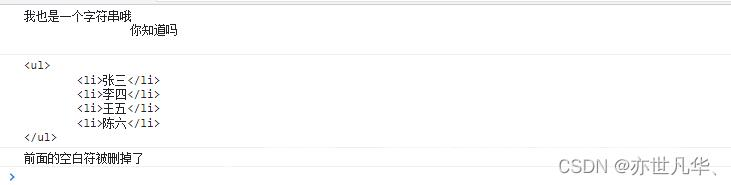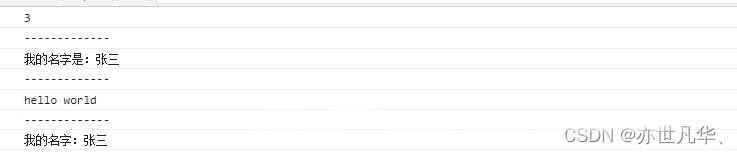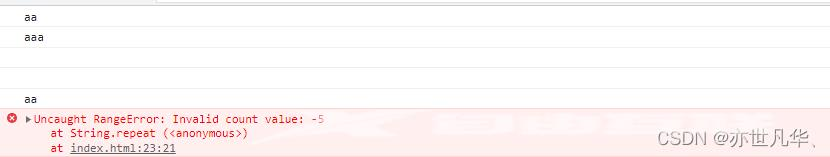﻿ ES6字符串和数值新增方法总结 - 自由资讯

# ES6字符串和数值新增方法总结

##### 目录
• 字符串新增方法
• 模板字符串
• 字符串对象新增方法
• 数值新增方法
• Math数值新增方法
• 总结

主要讲解了ES6中字符串与数值新增的常见的主要方法， 对其做了如下总结：

## 字符串新增方法

ES6对字符串新增的方法做一简单总结：

### 模板字符串

内容中的空格和换行都是可以被保留的

```<script>
let str = `我也是一个字符串哦
你知道吗
`
console.log(str);
let str1 = `<ul>
<li>张三</li>
<li>李四</li>
<li>王五</li>
<li>陈六</li>
</ul>`
console.log(str1);
//如果想删掉字符串的头尾空白符 可以使用 trim() 方法进行清除
let str2 = `    前面的空白符被删掉了`
console.log(str2.trim());
</script>```内容中的变量(可以是数值、函数、字符串、对象等)可以写在 \${} 之中：

```<script>
//数值运算
let a = 1
let b = 2
console.log(`\${a+b}`);
console.log('-------------');
//字符串拼接
let str = '张三'
let name1 = `我的名字是：\${str}`
console.log(name1);
console.log('-------------');
//函数使用
function fun(){
return "hello world"
}
console.log(`\${fun()}`);
console.log('-------------');
//对象使用
let obj = {
name:'张三'
}
console.log(`我的名字：\${obj.name}`);
</script>```### 字符串对象新增方法

在字符串中查找指定元素：includes()startWith()endsWith()

```<script>
//JS中只有 indexOf() 可以查找一个字符串中是否含特定元素，ES6提供三种方法
//includes()：返回布尔值，表示是否找到了参数字符串。
let str = 'Hello World'
console.log(str.includes('W'));//true

//startsWith()：返回布尔值，表示参数字符串是否在原字符串的头部。
let str1 = 'Hello People'
console.log(str1.startsWith('H'));//true

//endsWith()：返回布尔值，表示参数字符串是否在原字符串的尾部。只有endsWith()针对的是前n个字符，另外两种针对的是后n个字符
let str2 = 'Hello Animal'
console.log(str2.endsWith('l'));//true
</script>```

在字符串中将指定字符串重复n次：repeat()

```<script>
let str = 'a'
console.log(str.repeat(2));
//参数如果是小数就会取整，如果是0到-1之间的取整就是0
console.log(str.repeat(3.5));
console.log(str.repeat(-0.6)); //为 ""

//参数如果是NaN等同于0，如果是字符串先会转换为数字
console.log(str.repeat(NaN)); //为 ""
console.log(str.repeat('2'));

//参数如果是负数或者Infinity
console.log(str.repeat(-5));
console.log(str.repeat(Infinity));
</script>``````<script>
* 第一个参数是：字符串补全生效的最大长度
* 第二个参数是：用来补全的字符串,如果不写，默认就是字符串
*/
let str = 'aaa'

//如果原字符串大于或等于设置的最大长度，补充失效，输出原字符串

//如果补全字符内容超过规定最大长度，截去超出的长度
</script>```在字符串中指定字符串消除空白：trimStart()trimEnd()

```<script>
//trimStart()消除字符串头部的空格，trimEnd()消除尾部的空格
const str = '  hello   '
console.log(str.trimStart());//'hello   '
console.log(str.trimEnd());  //'   hello'
</script>```

在字符串中返回参数指定位置的字符：at()

```<script>
//at()支持负索引，如果参数超出了字符串范围则返回underfined
const str = 'hello'
console.log(str.at(2));  // l
console.log(str.at(-1)); //o
console.log(str.at(12)); //underfined
</script>```

在字符串中替换指定内容：replaceAll()

```<script>
//在字符串实例方法中，replace() 只能替换第一个字符,而ES引入的replaceAll()可以替换所有匹配
let str = 'aaabbedreaa'
console.log(str.replaceAll('a','_')); // ___bbedre__

//如果使用正则表达式，必须加上g修饰符
console.log(str.replaceAll(/a/g,'A')); // AAAbbedreAA

//replaceAll()的第二个参数是一个字符串，表示替换的文本，其中可以使用一些特殊字符串。
//\$&：匹配的字符串。
console.log('abbc'.replaceAll('b', '\$&')); // 'abbc'

//\$` ：匹配结果前面的文本。
console.log('abbc'.replaceAll('b', '\$`')); // 'aaabc'

//\$'：匹配结果后面的文本。
console.log('abbc'.replaceAll('b', `\$'`)); // 'abccc'

//\$n：匹配成功的第n组内容，n是从1开始的自然数。这个参数生效的前提是，第一个参数必须是正则表达式。
console.log('abbc'.replaceAll(/(ab)(bc)/g, '\$2\$1')); // 'bcab'

//\$\$：指代美元符号\$。
console.log('abc'.replaceAll('b', '\$\$')); // 'a\$c'
</script>```

## 数值新增方法

ES6对数值新增的方法做一简单总结：

在数值上提供判断数值是否有限、检查数值是否为NaN：Number.isFinite()Number.isNaN()

```<script>
// Number.isFinite()：判断数值是否有限，即不是Infinity；注意：参数类型不是数值，Number.isFinite一律返回false。
console.log(Number.isFinite(15)); //true
console.log(Number.isFinite(-5)); //true
console.log(Number.isFinite('hello')); //false
console.log(Number.isFinite(true)); //false

// Number.isNaN():用来检查一个值是否为NaN
console.log(Number.isNaN(NaN)); //true
console.log(Number.isNaN(9/NaN));//true
console.log(Number.isNaN(true));//false
console.log(Number.isNaN('hello'));//false
</script>```

在数值上提供判断数值是否为整数、整数是否落在安全整数范围之中：Number.isInteger()Number.isSafeInteger()

```<script>
// Number.isInteger()：用来判断一个数值是否为整数。如果对数据精度的要求较高，不建议使用
console.log(Number.isInteger(12)); //true
console.log(Number.isInteger(3.1)); //false
console.log(Number.isInteger(12.0)); //true  整数和浮点数采用的是同样的储存方法，所以 25 和 25.0 被视为同一个值。
console.log(Number.isInteger('12')); //false
console.log(Number.isInteger(3.0000000000000001)); //true 小数的精度达到了小数点后16个十进制位，转成二进制位超过了53个二进制位，导致最后的那个1被丢弃了。
console.log(Number.isInteger(5E-325)); //true 小于 JavaScript 能够分辨的最小值，会被自动转为 0

// Number.isSafeInteger()：则是用来判断一个整数是否落安全整数范围之内。
// JavaScript 能够准确表示的整数范围在-2^53到2^53之间（不含两个端点），超过这个范围，无法精确表示这个值。
// ES6 引入了Number.MAX_SAFE_INTEGER和Number.MIN_SAFE_INTEGER这两个常量，用来表示这个范围的上下限
Number.MAX_SAFE_INTEGER === Math.pow(2, 53)
Number.MIN_SAFE_INTEGER === -Math.pow(2, 53)
console.log(Number.isSafeInteger(3)); //true
console.log(Number.isSafeInteger(3.1)); //false
console.log(Number.isSafeInteger(Number.MAX_SAFE_INTEGER)); //true
</script>```

在数值上取整数与浮点数：Number.parseInt()Number.parseFloat()

```<script>
// Number.parseInt
console.log(Number.parseInt('12.45')); // 12
// Number.parseFloat
console.log(Number.parseFloat('12.45'));// 12.45
</script>```

在数值上新增极小常量设置误差范围：Number.EPSILON

```<script>
// Number.EPSILON的实质是：一个可以接受的最小误差范围。
function withinErrorMargin (left, right) {
return Math.abs(left - right) < Number.EPSILON * Math.pow(2, 2);
}
console.log(0.1 + 0.2 === 0.3); // false
console.log(withinErrorMargin(0.1 + 0.2, 0.3));// true

console.log(1.1 + 1.3 === 2.4);// false
console.log(withinErrorMargin(1.1 + 1.3, 2.4));// true
</script>```

### Math数值新增方法

在数值上去除小数部分返回整数部分：Math.trunc()

```<script>
// Math.trunc方法用于去小返整，非数值先转数值，不能转的返回NaN
console.log(Math.trunc(12.36));//12
console.log(Math.trunc(-0.12));//-0
console.log(Math.trunc(true));//1
console.log(Math.trunc(false));//0
console.log(Math.trunc('123.36'));//123
console.log(Math.trunc('fun')); //对于空值和无法截取整数的值，返回NaN。
</script>```

在数值上判断一个数是正数、负数、还是0：Math.sign()

```<script>
// 正数返回+1、负数返回-1、0或-0返回0或-1、负数返回-1、0或-0返回0或-0、其他值返回NaN
console.log(Math.sign(12)); //1
console.log(Math.sign(-12)); //-1
console.log(Math.sign(0)); //0
console.log(Math.sign(-0)); //-0
console.log(Math.sign('sign')); //NaN
</script>```

在数值上计算一个数的立方根：Math.cbrt()

```<script>
// 对于非数值先转换为数值，转换不了的返回NaN
console.log(Math.cbrt(8)); //2
console.log(Math.cbrt('8')); //2
console.log(Math.cbrt('hello')); //NaN
</script>```

在数值上返回所有参数平方和的平方根：Math.hypot()

```<script>
// 例如：3 的平方加上 4 的平方，等于 5 的平方。
console.log(Math.hypot(3,4)); // 5
// 如果参数不是数值，Math.hypot方法会将其转为数值。只要有一个参数无法转为数值，就会返回 NaN。
console.log(Math.hypot(6,'8')); // 10
console.log(Math.hypot(3,4,'sum')); //NaN
</script>```

在数值上ES6新增四个对数方法：Math.expml()Math.log1p()Math.log10()Math.log2()

```<script>
// Math.expm1(x)返回 e^x - 1
console.log(Math.expm1(0));//0 e^0-1=0
console.log(Math.expm1(1));//1.718281828459045

// Math.log1p(x)方法返回1 + x的自然对数，即Math.log(1 + x)。如果x小于-1，返回NaN。
console.log(Math.log1p(1));// 0.6931471805599453
console.log(Math.log1p(0));// 0
console.log(Math.log1p(-1));// -Infinity
console.log(Math.log1p(-2));// NaN

// Math.log10(x)返回以 10 为底的x的对数。如果x小于 0，则返回 NaN。
console.log(Math.log10(2));// 0.3010299956639812
console.log(Math.log10(1));// 0
console.log(Math.log10(0));// -Infinity
console.log(Math.log10(-2));// NaN

// Math.log2(x)返回以 2 为底的x的对数。如果x小于 0，则返回 NaN。
console.log(Math.log2(3));// 1.584962500721156
console.log(Math.log2(2));// 1
console.log(Math.log2(1));// 0
console.log(Math.log2(-2));// NaN
</script>```

## 总结

到此这篇关于ES6字符串和数值新增方法的文章就介绍到这了,更多相关ES6字符串和数值新增方法内容请搜索自由互联以前的文章或继续浏览下面的相关文章希望大家以后多多支持自由互联！

上一篇：微信小程序实现简易计算器功能
下一篇：没有了
网友评论
相关栏目
﻿